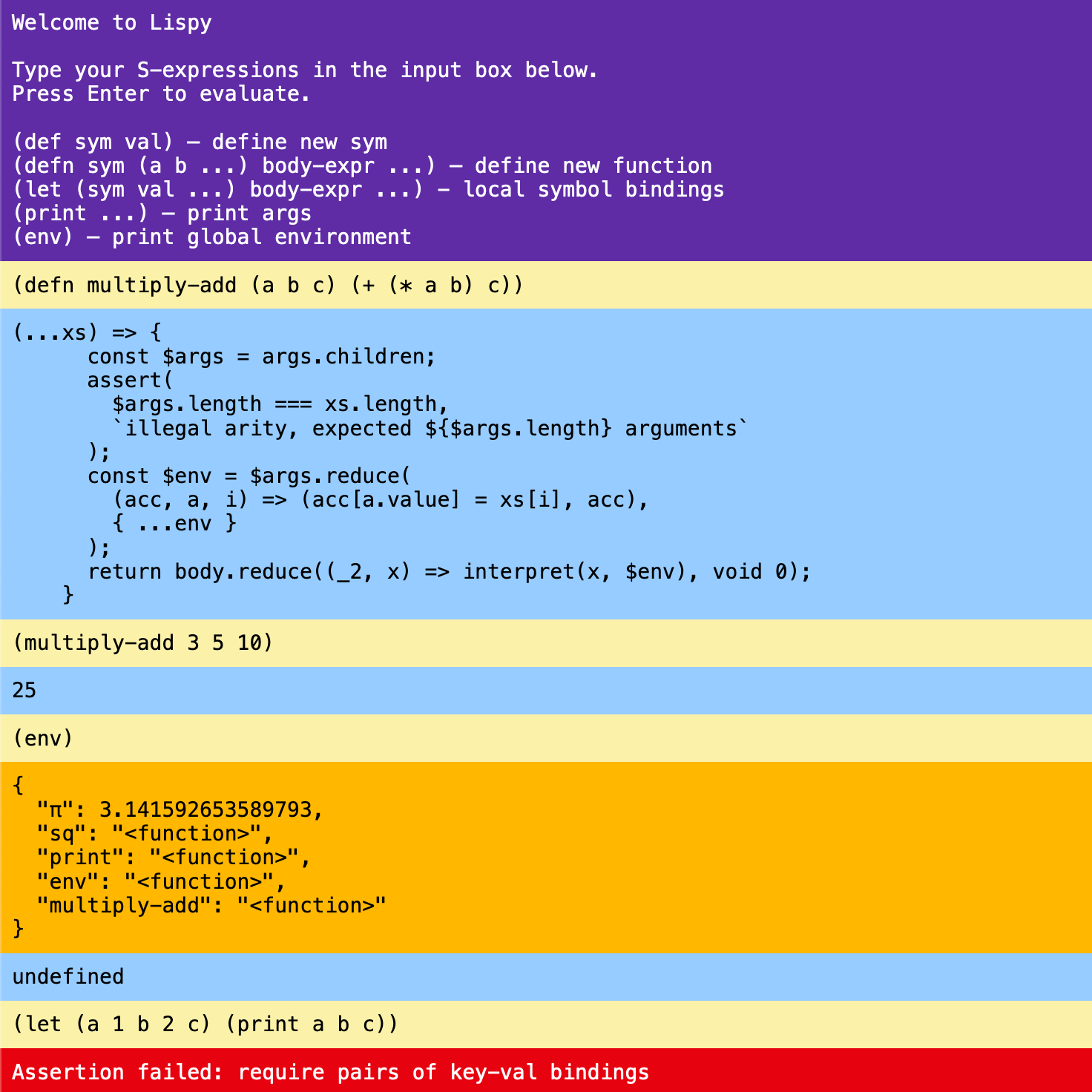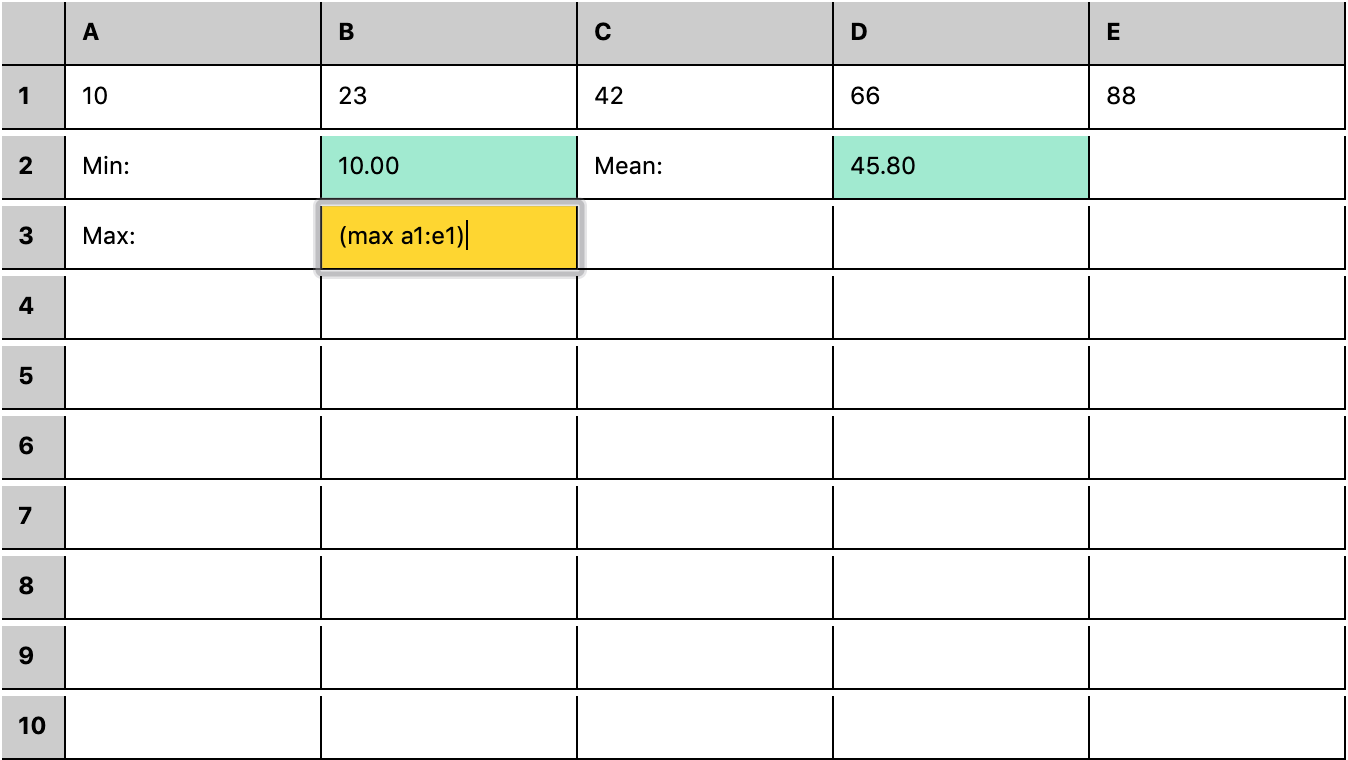## @thi.ng/sexpr0.5.0 • Public • Published

#This project is part of the @thi.ng/umbrella monorepo and anti-framework.

Basic, but configurable and extensible S-Expression tokenizer, parser, AST builder and runtime / interpreter skeleton for custom, sandboxed DSL implementations.

The following default syntax rules are used:

• whitespace: space, tab, newline, comma
• expression delimiters: (, )
• numbers: any float notation valid in JS, hex ints prefixed w/ 0x
• string delimiters: "
• line comments: ;

Everything else is parsed as is, i.e. as symbol.

## Status

ALPHA - bleeding edge / work-in-progress

Search or submit any issues for this package

## Related packages

• @thi.ng/parse - Purely functional parser combinators & AST generation for generic inputs

## Installation

yarn add @thi.ng/sexpr

ES module import:

<script type="module" src="https://cdn.skypack.dev/@thi.ng/sexpr"></script>

Skypack documentation

For Node.js REPL:

const sexpr = await import("@thi.ng/sexpr");

Package sizes (brotli'd, pre-treeshake): ESM: 771 bytes

## Usage examples

Several demos in this repo's /examples directory are using this package.

A selection:

Screenshot Description Live demo SourceBrowser REPL for a Lispy S-expression based mini language Demo Sourcerstream based spreadsheet w/ S-expression formula DSL Demo Source

## API

Generated API docs

### Tokenize only (iterator)

The tokenize function returns an iterator of tokens incl. location details. Any whitespace is skipped and whitespace characters are configurable.

[...tokenize((* (+ 3 5) 10))];
// [
//   { value: '(', line: 0, col: 0 },
//   { value: '*', line: 0, col: 1 },
//   { value: '(', line: 0, col: 3 },
//   { value: '+', line: 0, col: 4 },
//   { value: '3', line: 0, col: 6 },
//   { value: '5', line: 0, col: 8 },
//   { value: ')', line: 0, col: 9 },
//   { value: '10', line: 0, col: 11 },
//   { value: ')', line: 0, col: 13 }
// ]

### AST generation

The parse function takes a source string or iterable of tokens and parses it into an AST.

parse(tokenize((* (+ 3 5) 10)));
// or directly from string
parse((* (+ 3 5) 10));
{
"type": "root",
"children": [
{
"type": "expr",
"value": "(",
"children": [
{
"type": "sym",
"value": "*"
},
{
"type": "expr",
"value": "(",
"children": [
{
"type": "sym",
"value": "+"
},
{
"type": "num",
"value": 3
},
{
"type": "num",
"value": 5
}
]
},
{
"type": "num",
"value": 10
}
]
}
]
}

### Interpreter

The below code example implements a small Lisp-like language, complete with interpreter and can be used an the basis for an easily extensible (and more optimized) setup. Even this barebones implementation allows for defining of symbols & functions, lexical scoping, FFI via providing externally defined functions via the global environment, variadic math ops...

import type { Fn, Fn2 } from "@thi.ng/api";
import { DEFAULT, defmulti, type MultiFn2 } from "@thi.ng/defmulti";
import {
parse, runtime,
type ASTNode, type Expression, type Implementations, type Sym
} from "@thi.ng/sexpr";

// evaluator: parses given source string into an abstract syntax tree (AST) and
// then recursively executes all resulting AST nodes using the
// interpret() function defined below. Returns the result of the last node.
const $eval = (src: string, env: any = {}) => parse(src).children.reduce((_, x) => interpret(x, env), <any>undefined); // build runtime (interpreter) w/ impls for all AST node types. the generics are // the types of the custom environment and the result type(s) of expressions. // this is a multiple-dispatch function (see thi.ng/defmulti) which chooses // implementations based on the AST node type const interpret = runtime<Implementations<any, any>, any, any>({ // for expression nodes (aka function calls) delegate to builtins // (implementations are defined further below) expr: (x, env) => builtins(x.children, env), // lookup symbol's value (via its name) in environment sym: (x, env) => env[x.value], // strings and numbers evaluate verbatim str: (x) => x.value, num: (x) => x.value, }); // another multiple-dispatch function for DSL builtins. we will call this // function for each S-expression node and it will delegate to the actual impl // based on the expression's first item (i.e. a symbol/fn name) const builtins: MultiFn2<ASTNode[], any, any> = defmulti((x) => x.value); // helper function which interprets all given AST nodes and returns an array of // their result values const evalArgs = (nodes: ASTNode[], env: any) => nodes.map((a) => interpret(a, env)); // helper function for basic math ops variable arity. // with 2+ args: (+ 1 2 3 4) => 10 // and special cases for 1 arg only, i.e. // (+ 2) => 0 + 2 => 2 // (- 2) => 0 - 2 => -2 // (* 2) => 1 * 2 => 2 // (/ 2) => 1 / 2 => 0.5 const mathOp = (fn: Fn2<number, number, number>, fn1: Fn<number, number>) => ([_, ...args]: ASTNode[], env: any) => { const first = interpret(args, env); return args.length > 1 ? // use a reduction for 2+ args evalArgs(args.slice(1), env).reduce((acc, x) => fn(acc, x), first) : // apply special case unary function fn1(first); }; // implementations of built-in core functions builtins.addAll({ "+": mathOp((acc, x) => acc + x, (x) => x), "*": mathOp((acc, x) => acc * x, (x) => x), "-": mathOp((acc, x) => acc - x, (x) => -x), "/": mathOp((acc, x) => acc / x, (x) => 1 / x), // count returns the length of first argument (presumably a string) // (e.g. (count "abc") => 3) count: ([_, arg], env) => interpret(arg, env).length, // concatenates all args into a space-separated string and prints it // returns undefined print: ([_, ...args], env) => console.log(evalArgs(args, env).join(" ")), // defines as new symbol with given value, stores it in the environment and // then returns the value, e.g. (def magic 42) def: ([_, name, value], env) => (env[(<Sym>name).value] = interpret(value, env)), // defines a new function with given name, args and body, stores it in the // environment and returns it, e.g. (defn madd (a b c) (+ (* a b) c)) defn: ([_, name, args, ...body], env) => { // create new vararg function in env return (env[(<Sym>name).value] = (...xs: any[]) => { // create new local env with arguments bound to named function args // (i.e. simple lexical scoping) const$env = (<Expression>args).children.reduce(
(acc, a, i) => ((acc[(<Sym>a).value] = xs[i]), acc),
{ ...env }
);
// execute function body with local env, return result of last expr
return body.reduce((_, x) => interpret(x, $env), <any>undefined); }); }, // add default/fallback implementation to allow calling functions defined in // the environment (either externally or via defn) [DEFAULT]: ([x, ...args]: ASTNode[], env: any) => { const name = (<Sym>x).value; const f = env[name]; if (!f) throw new Error(missing impl for:${name});
return f.apply(null, evalArgs(args, env));
},
});

// testing our toy Lisp DSL...

// define symbol and use in another expression
$eval((def chars "abc") (print (count chars) "characters")); // 3 characters // define function (multiply-add) and test$eval((defn madd (a b c) (+ (* a b) c)) (print (madd 3 5 (* 5 2))));
// 25

// pre-define function via environment, then use in DSL
$eval((print (join " | " (+ 1 2) (* 3 4) (- 5 6) (/ 7 8))), { join: (sep: string, ...xs: any[]) => xs.join(sep), }); // 3 | 12 | -1 | 0.875 // testing lexical scoping... // function foo has a as local (with precedence over global) binding, // but function bar correctly uses binding of the outer env, even if // called from foo...$eval(
(def a 1)
(defn foo (a) (print "foo" a "bar" (bar)))
(defn bar () (+ a 1))

(print "root #1" a)
(foo 100)
(print "root #2" a)
);
// root #1 1
// foo 100 bar 2
// root #2 1

See test/ for a more in-depth version of this example...

### Custom syntax

import { parse } from "@thi.ng/sexpr";

// define syntax overrides (keep default whitespace rules)
const syntax = {
scopes: [["<", ">"], ["{", "}"]],
string: "'"
};

console.log(JSON.stringify(parse(<nest { a '2' b 3 }>, syntax), null, 2));

Resulting JSON output:

{
"type": "root",
"children": [
{
"type": "expr",
"value": "<",
"children": [
{
"type": "sym",
"value": "nest"
},
{
"type": "expr",
"value": "{",
"children": [
{
"type": "sym",
"value": "a"
},
{
"type": "str",
"value": "2"
},
{
"type": "sym",
"value": "b"
},
{
"type": "num",
"value": 3
}
]
}
]
}
]
}

## Authors

If this project contributes to an academic publication, please cite it as:

@misc{thing-sexpr,
title = "@thi.ng/sexpr",
author = "Karsten Schmidt",
note = "https://thi.ng/sexpr",
year = 2019
}

## Keywords

### Repository

github.com/thi-ng/umbrella

215

0.5.0

Apache-2.0

40.2 kB

14